## Example Questions

### Example Question #1 : How To Find The Sine Of An AngleWhat is the sine of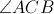?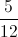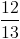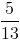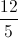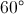Explanation:

Sine can be found using the SOH CAH TOA method. For sine we do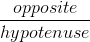.

### Example Question #1 : How To Find The Sine Of An Angle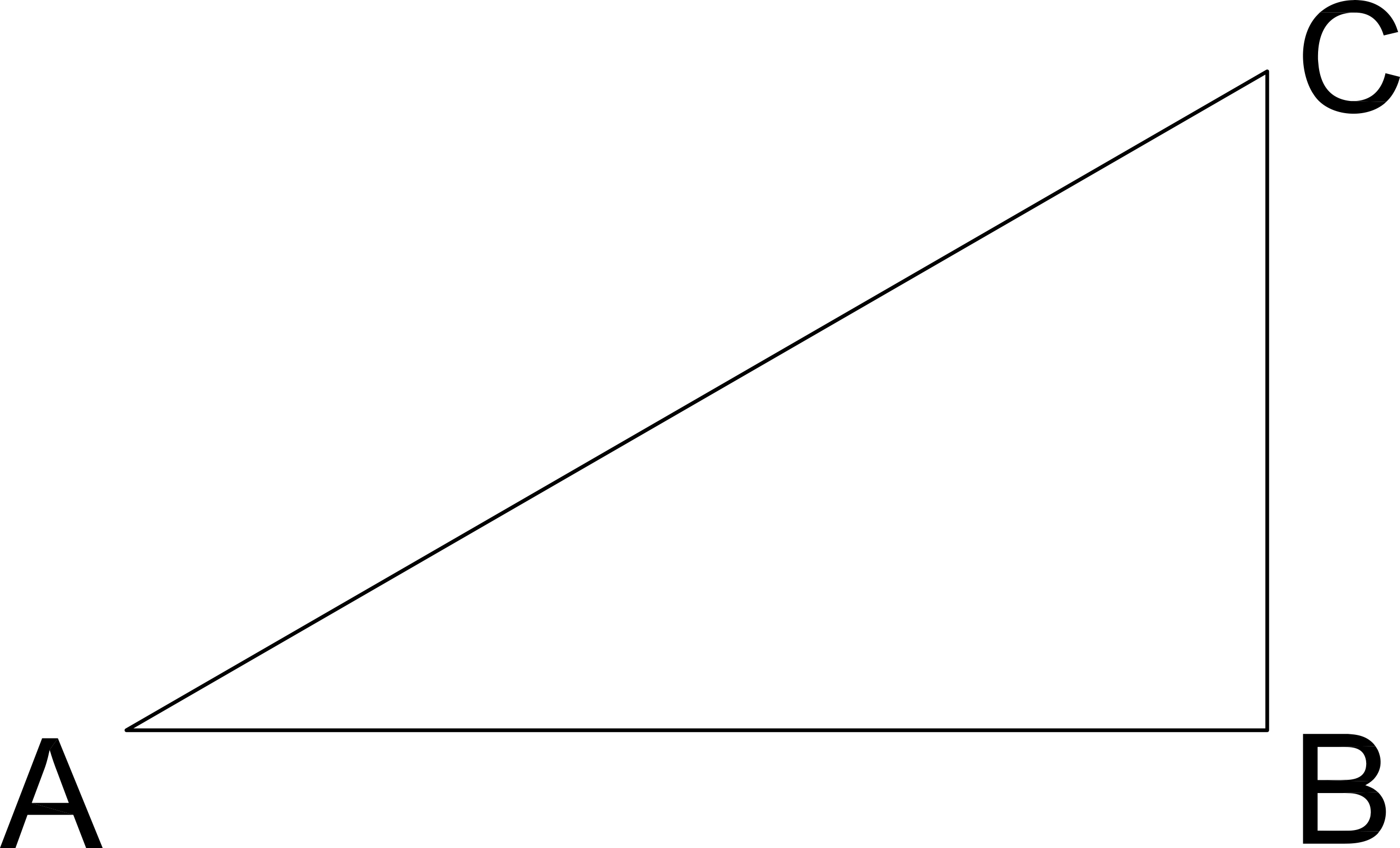See right triangle ABC.  If the length AB is 8 and the length of BC is 6, what is the sine of angle A?

1

0.8

10

6

0.6

0.6

Explanation:

Sine A = Opposite / Hypotenuse = BC / AC

To find AC, use Pythagorean Theorum

AB2 + BC2 = AC2

82 + 62 = AC2

64 + 36 = AC2

100 = AC2

AC = 10

Sine A = BC / AC = 6 / 10 = 0.6

### Example Question #1 : How To Find The Sine Of An Angle

Solve for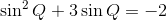over the interval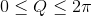Q = π or 3π 2 2

Q = 3π or does not exist 2

Q = π or 2π

Q = π or does not exist 2

Q = 3π or does not exist 2

Explanation:

Substitute x = sinQ and solve the new equation x2 + 3x = –2 by factoring.  Be sure to change variables back to Q.  As a result, sinQ = –1 or sinQ = –2.  This function is bounded between –1 and 1 so sinQ can never be –2 and sinQ is –1 only at 3π/2 or 270 °.

### Example Question #4 : How To Find The Sine Of An Angle

If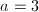,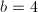, and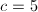, what is the sine of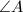?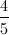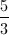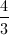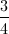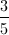Explanation:

Recall that sin = opposite / hypotenuse.  Based on the figure shown, we see thatis the opposite side needed andis the hypotenuse.  Plug these values in to solve.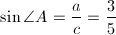### Example Question #5 : How To Find The Sine Of An AngleTriangle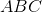shown is a right triangle. If line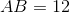and line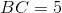, what is the sine of the angle at?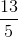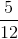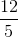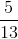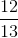Explanation: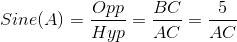Now solve for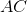using Pythagorean Theorem: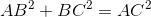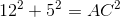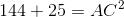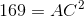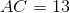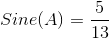### Example Question #6 : How To Find The Sine Of An Angle

If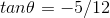, and if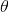is an angle betweenanddegrees, which of the following equals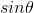?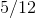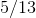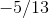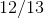Explanation:

An angle betweenanddegrees means that the angle is located in the second quadrant.

The tangent function is derived from taking the side opposite to the angle and dividing by the side adjacent to the angle (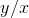, as shown in the image).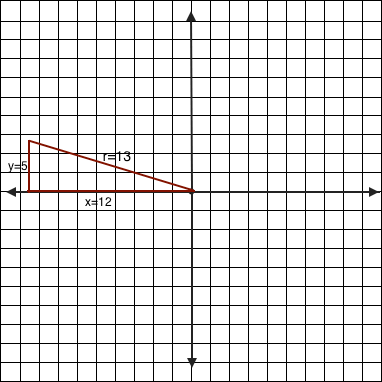Hence, the sideisunits long and sideisunits high. Therefore, according to Pythagorean Theorem rules, the sidemust beunits long (since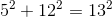).

The sine function is positive in the second quadrant. It is also equivalent to the side opposite the angle () divided by the hypotenuse ().

This makes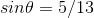.

### Example Question #7 : How To Find The Sine Of An Angle

A sine function has a period of, a-intercept of, an amplitude ofand no phase shift. These describe which of these equations?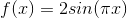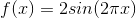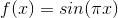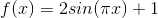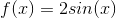Explanation:

Looking at this form of a sine function: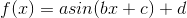We can draw the following conclusions:

•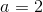because the amplitude is specified as.
•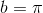because of the specified period ofsince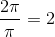.
•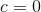because the problem specifies there is no phase shift.
•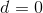because the-intercept of a sine function with no phase shift is.

Bearing these in mind,is the only function that fits all four of those.